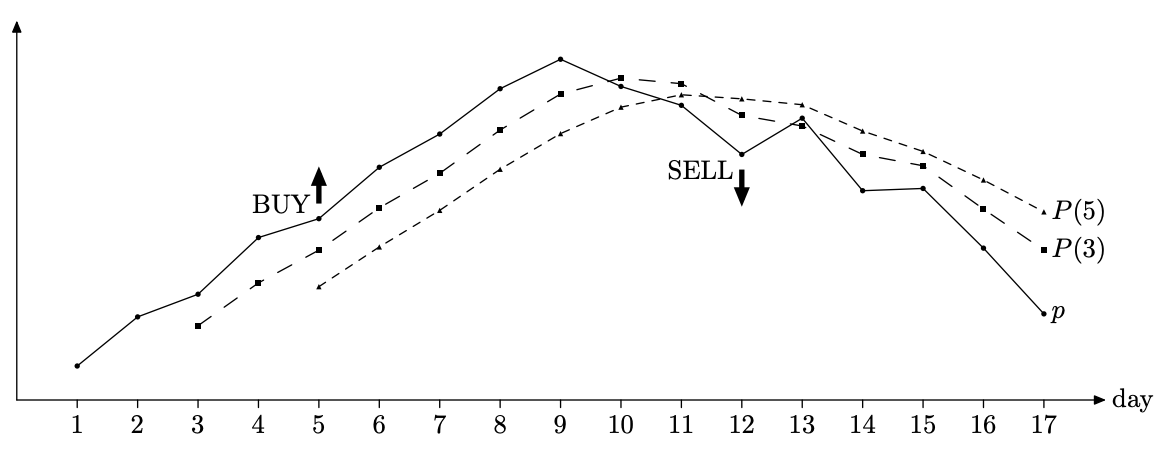시간 제한 메모리 제한 제출 정답 맞은 사람 정답 비율
2 초 128 MB 0 0 0 0.000%

## 문제

Frank is a professional stock trader for Advanced Commercial Markets Limited (ACM Ltd). He likes "easy trading" --- using a straightforward strategy to decide when to buy stock and when to sell it.

Frank has a database of historical stock prices for each day. He uses two integer numbers $m$ and $n$ ($1 \le m < n \le 100$) as parameters of his trading strategy. Every day he computes two numbers: $P(m)$ --- an average stock price for the previous $m$ days, and $P(n)$ --- an average stock price for the previous $n$ days. $P(m) > P(n)$ is an indicator of the upward trend (traders call it bullish trend), and $P(m) < P(n)$ is an indicator of the downward trend (traders call it bearish trend). In practice the values for $P(m)$ and $P(n)$ are never equal.

When a trend reverses from bearish to bullish it is a signal for Frank to buy stock. When a trend reverses from bullish to bearish it is a signal to sell.Frank has different values for $m$ and $n$ in mind and he wants to backtest them using historical prices. He takes a set of $k$ ($n < k \le 10\,000$) historical prices $p_i$ ($0 < p_i < 100$ for $1 \le i \le k$). For each $i$ ($n \le i \le k$) he computes $P_i(m)$ and $P_i(n)$ --- an arithmetic average of $p_{i-m+1} \dots p_i$ and $p_{i-n+1} \dots p_i$ respectively.

Backtesting generates trading signals according to the following rules.

• If $P_i(m) > P_i(n)$ there is a bullish trend for day $i$ and a "BUY ON DAY $i$" signal is generated if $i=n$ or there was a bearish trend on day $i-1$.
• If $P_i(m) < P_i(n)$ there is a bearish tread for day $i$ and a "SELL ON DAY $i$" signal is generated if $i=n$ or there was a bullish trend on day $i-1$.

Your task is to write a program that backtests a specified strategy for Frank --- you shall print a signal for the first tested day (day $n$) followed by the signals in increasing day numbers.

## 입력

The first line of the input file contains three integer numbers $m$, $n$, and $k$. It is followed by $k$ lines with stock prices for days 1 to $k$. Each stock price $p_i$ is specified with two digits after decimal point. Prices in the input file are such that $P_i(m) \ne P_i(n)$ for all $i$ ($n \le i \le k$).

## 출력

Write to the output file a list of signals --- one signal on a line, as described in the problem statement.

## 예제 입력 1

3 5 17
8.45
9.10
9.40
10.15
10.40
11.08
11.52
12.12
12.51
12.15
11.90
11.25
11.73
10.77
10.80
10.01
9.14


## 예제 출력 1

BUY ON DAY 5
SELL ON DAY 12